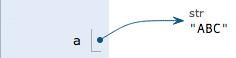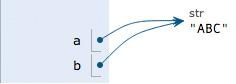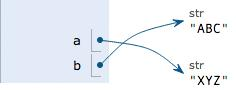# Python中的变量

## Python中的变量

2016-09-05 作者: zhangxiang 浏览: 1689 次

### 变量

a = 1

t_007 = 'T007'

Answer = True

a = 123
print (a)
a = 'ABC'
print (a)

int a = 123; // a是整数类型变量
a = "ABC"; // 错误：不能把字符串赋给整型变量

x = 10
x = x + 2

a = 'ABC'

1.在内存中创建了一个'ABC'的字符串；

2.在内存中创建了一个名为a的变量，并把它指向'ABC'。

a = 'ABC'
b = a
a = 'XYZ'
print (b)